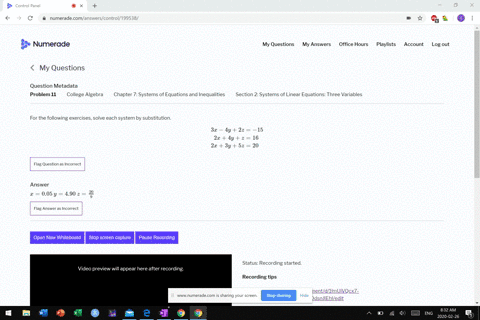Enroll in one of our FREE online STEM bootcamps. Join today and start acing your classes!View Bootcamps05:55
MB
Problem 10

# For the following exercises, determine whether the ordered triple given is the solution to the system of equations.$$\begin{array}{l}{-x-y+2 z=3} \\ {5 x+8 y-3 z=4 \quad \text { and }(4,1,-7)} \\ {-x+3 y-5 z=-5}\end{array}$$

## Discussion

You must be signed in to discuss.

## Video Transcript

So is 41 negative seven A solution Thio system of equations. So it needs to make all three equations true. All right. Makes any of them not true will be dumped The problem. Go ahead with this goddamn door. Substitution says so. Foreign for acts will get negative Minus one waas. Two times, maybe seven. We want to know. Is that three? So negative floor minus one is negative. Five negative. Five bus Negative 14. It's not equal three. So this is not a solution. Do the system.